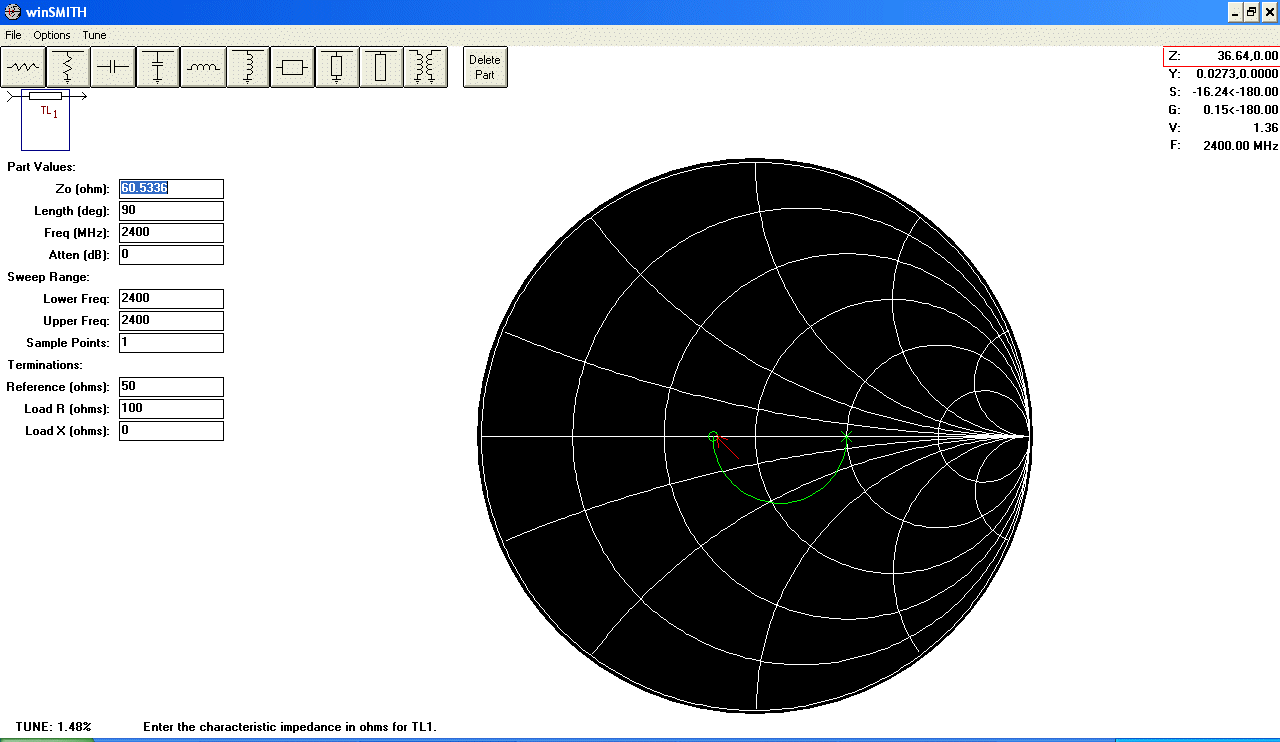How to measure the impedance of a microstrip Transmission Line

Iulian Rosu, YO3DAC / VA3IUL

http://www.qsl.net/va3iul

This is a procedure how to measure the unknown impedance of a microstrip Transmission Line printed on a PCB.

For this test you need a Network Analyzer and a Smith Chart software as WinSMITH.

To measure the impedance at a specific frequency is recommended that the length of the microstrip TL to be longer than a quarter wavelength (λ/4 or 90º). For example longer than 31 mm at 2.4GHz.

1. If the printed microstrip TL has a connector connected to one end (SMA or whatever) go to step nr 6.
2. If the microstrip Transmission Line do not have a connector attached to one end (the TL is in the middle of the PCB) you have to use for the measurement a thin 50 ohms coax cable (flexible or semi-rigid) which has an SMA connector attached to one end.
3. Calibrate the Network Analyzer for One Port Reflection using this thin 50 ohms cable. For calibration connect the SMA connector to the Network Analyzer, and for the other end of the coax cable use nothing for OPEN, solder the center with the shield for SHORT, and solder two 100 ohms resistor in parallel (resulting 50 ohms) for LOAD. The reason soldering two resistors in parallel is to minimize the parasitic inductance of built load.
4. After calibration do a quick check measuring Return Loss (S11) of this cable having the two parallel 100 ohms resistors connected. The Return Loss should be better than -30dB, otherwise the quality of the coax cable that you are using is poor and you have to replace it.
5. Remove the two 100 ohms resistors and connect the coax cable to one end of your TL printed on the board.
6. At the other end of the TL solder to the ground a 100 ohms SMD resistor.
7. Using the Network Analyzer (Smith Chart format) measure S11 of the line. On the Smith Chart you can see a half-circle if the line is λ/4 (90º) or a full circle if is λ/2 (180º).
8. Using the Analyzer Marker read the Z impedance of the line where the circle (or half-circle) is crossing the symmetry axe of the Smith Chart (left side, opposite of the 100 ohms reference).
9. The reading for a perfect 50 ohms Transmission Line will be 25 ohms. If the reading is different than 25 ohms means the TL is not 50 ohms.
10. Open WinSMITH and insert a series Transmission Line. Set the operating frequency. Set 50 ohms for Reference. Set 100 ohms for Load R. Set 0 ohms for Load X. Set Length to 90º.11. Highlight the Zo value (default is 50 ohms). Point the mouse cursor on the symmetry axe until you read Z on the up-right corner equal to the value you read on the Network Analyzer.
12. Tune Zo using PageUp and PageDown keys, until the circle reach the impedance pointed with the cursor. This is the actual impedance of the printed Transmission Line.
13. For example if you read on the Network Analyzer a value of 36 ohms (left side of the semi-circle where intersect the symmetry axe) means the impedance of the printed Transmission Line is 60 ohms.home